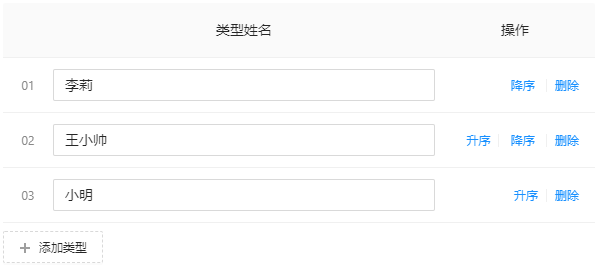# antdv table 数据操作升序、降序、删除、增加功能_xujing_06_antd通过前端控制升序和降序

let newData: any[] = [];1、升序 主要代码newData.filter((item, index) =&gt; { if (item.key == data.key) { newData[index] = newData.splice(index - 1, 1, newData[index]); }});state.dataSource = newData;2、降序 主要代码（newData[index] = n......`let newData: any[] = [];`

1、升序--向上移动一格?主要代码

```newData.filter((item, index) => {
if (item.key == data.key) {
newData[index] = newData.splice(index - 1, 1, newData[index]);
}
});```
`state.dataSource = newData;`

2、降序 --向下移动一格 主要代码（newData[index] = newData.splice(index+1, 1, newData[index]);这种方式table报错）

```newData.filter((item, index) => {
if (item.key == data.key) {
newData.splice(index, 1);
newData.splice(index + 1, 0, data);
}
});```
`state.dataSource = newData;`

3、删除

```newData = state.dataSource.filter(item => item.key !== data.key);
state.dataSource = newData;
```

4、增加

```let length = state.dataSource.length;
length++;
const newObj: { key: string; name: string } = {
key: `\${length}`,
name: '',
};
state.dataSource.push(newObj);```